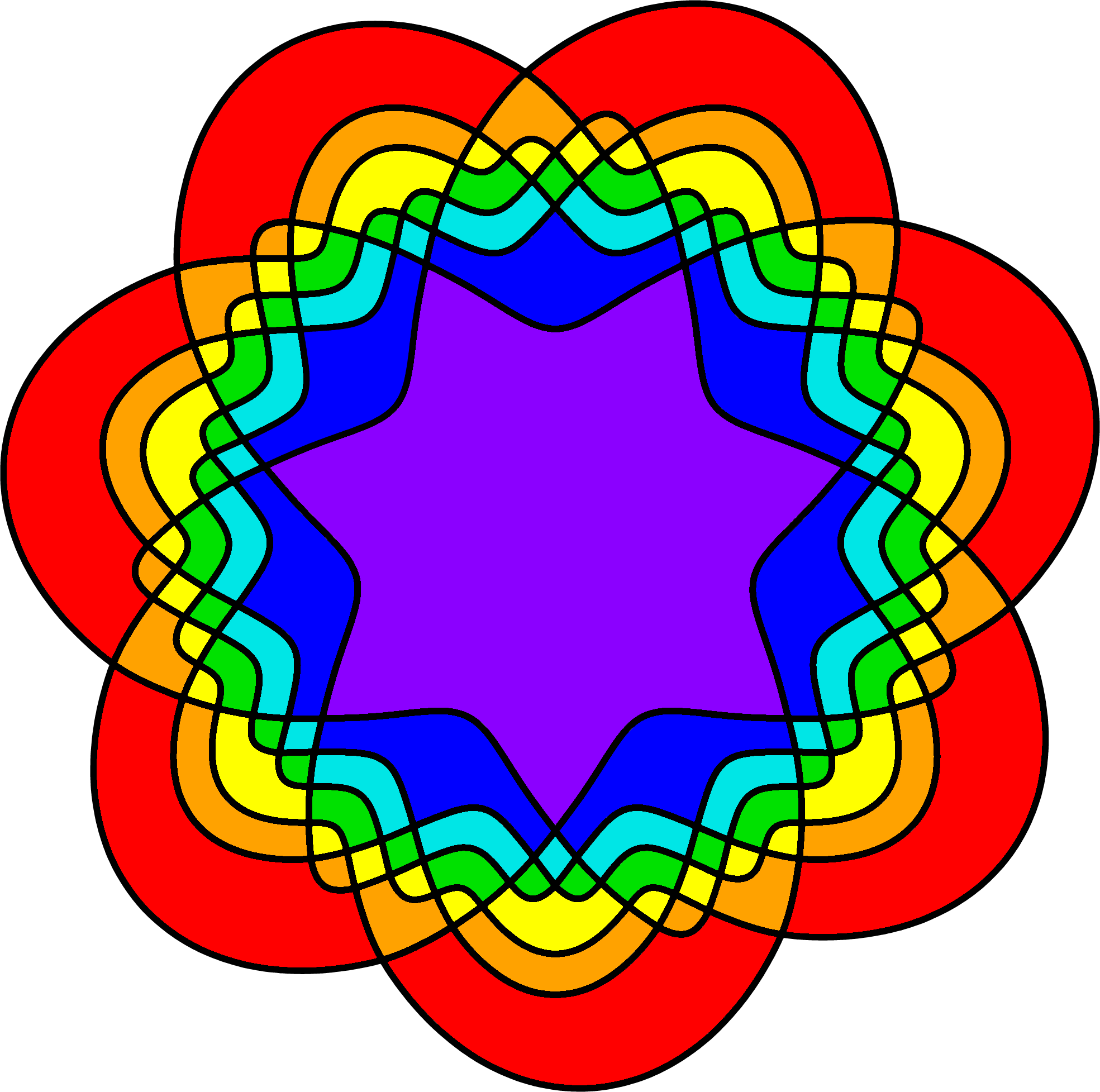# A New Rose : The First Simple Symmetric 11-Venn Diagram

Khalegh Mamakani, Department of Computer Science, University of Victoria, Canada.
Frank Ruskey, Department of Computer Science, University of Victoria, Canada.

### Abstract:

In this paper we introduce a new property of Venn diagrams called crosscut symmetry. Utilizing a computer search restricted to crosscut symmetry we found many simple symmetric 11-Venn diagrams. This answers a problem that has been open since the 1960's. The first such diagram that was discovered is shown here.

### Explanatory Examples with 7 Curves

Below are two symmetric 7-Venn diagrams. A crosscut is a curve segment that intersects every other curve sequentially without repetition. The one on the left, "M11", has a crosscut, but it is not crosscut symmetric. The one on the right, "M01", does not have a crosscut.Below are two symmetric 7-Venn diagrams. The one on the left, "Hamilton", is both polar symmetric and crosscut symmetric. The one on the right, "M4", is crosscut symmetric, but it is not polar symmetric. M4 is the one that is used for illustrative purposes in the paper.Crosscut symmetry refers to the idea that the regions on either side of the crosscut can be drawn with a bilateral symmetry. The diagram below shows what we call a "cluster"; the colored part of the diagram on the left. The central portion of the cluster shows the bilateral symmetry; the colored part of the diagram on the right. The diagram shown is "M4".### Examples with the First 11 Curve Venn Diagram

It is recommended that you view these on a big screen! Also, if some of the diagrams are not showing or are clipped, then you should try using a browser such as Firefox or Chrome. The illustration below shows a cyclindrical representation of the 11-Venn diagram.And here is 1/11th of the cylindrical representation.Below is the diagram drawn on the plane, with two different styles of filling.And here is a blow up of part of Newroz.The illustration below shows the 11-Venn diagram with one curve highlighted (shown in white).Below we show 1/11th of the cylindrical representation of the diagram, drawn with the cross cut drawn as a vertical line segment on the left and right as it intersects the other curves, and with the bilateral symmetry made explicit.